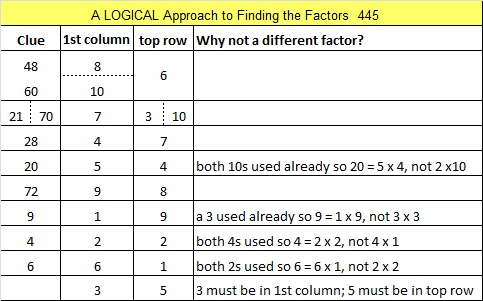# 445 and Level 4

Supposedly there is something interesting about every number. What is interesting about the number 445? When you write it in base 9, the digits are reversed. How do you convert 445 from base 10 to base 9?

Solution: The powers of 9 less than 445 in descending order are 81, 9, and 1. We first divide 445 by 81, next we divide the remainder by 9, and lastly, we divide that remainder by 1 as illustrated below.Thus 445 (base 10) = 544 (base 9).Print the puzzles or type the factors on this excel file: 10 Factors 2015-03-30

• 445 is a composite number.
• Prime factorization: 445 = 5 x 89
• The exponents in the prime factorization are 1 and 1. Adding one to each and multiplying we get (1 + 1)(1 + 1) = 2 x 2 = 4. Therefore 445 has exactly 4 factors.
• Factors of 445: 1, 5, 89, 445
• Factor pairs: 445 = 1 x 445 or 5 x 89
• 445 has no square factors that allow its square root to be simplified. √445 ≈ 21.0950445 is the hypotenuse of four Pythagorean triples:

• [267-356-445] which is [3-4-5] times 89
• [195-400-445] which is [39-80-89] times 5
• Primitive [84-437-445] (Note: 84 = 2(2 x 21) and 437 = 21^2 – 2^2, while 445 = 21^2 + 2^2)
• and Primitive [203-396-445] (Note: 396 = 2(18 x 11) and 203 = 18^2 – 11^2, while 445 = 18^2 + 11^2)## 11 thoughts on “445 and Level 4”

1.Joseph Nebus

Oh, that is a neat coincidence. I wonder what some other similar ones might be.

•ivasallay

This is a topic I’ve never taken the time to explore. It could be fun to find some other similar ones.

2.abyssbrain

Haha, the base 25 of 445 is HK (or Hong Kong) and its base 24 is ID, so its like HKID

•ivasallay

Can you do these other base calculations without paper and pencil, too?

•abyssbrain

For up to base 16 I can do that in my head, but anything higher than that, I usually write my partial answers on a paper to reduce the mental strain.

•ivasallay

That’s pretty amazing. If I wanted to express 445 in base 2 or 3, I would definitely use paper and pencil. Both of them have too many powers less than 445. I would have a difficult time remembering the quotients and remainders without writing them down.

•abyssbrain

I actually use a different method for converting bases. E.g. 445 in base 9

445/9 = 49 r. 4
49/9 = 5 r. 4
So, it’s equal to 544

I’m also used to doing mental divisions for divisors from 2 to 15, I can do 16 to 19 with some difficulty.

3.ivasallay

Okay, there’s more than one way to do the problem, and your way doesn’t require a person to know any powers. Probably most people would prefer your way because of that.

•abyssbrain

I don’t know why, but many people find it more intuitive to convert other bases to base 10 using the way that you have shown (well in reverse)

e.g. 544 base 9 to base 10

5*(9^2) + 4*(9^1) + 4*(9^0) = 445

•ivasallay

The method I used definitely appeals to my intuition whether I’m converting to or from base 10. I learned some confusing way in school to change basis, and I don’t remember exactly what that method was, only that it was confusing.

•abyssbrain

Yeah, I remembered that during my 6th grade (I think), they taught this really strange method for converting to base 2 and 8. I didn’t even recall the method at all. That time, they also taught a very stupid and inefficient method for extracting square roots haha. Regarding my math studies, I hated that year the most.

This site uses Akismet to reduce spam. Learn how your comment data is processed.